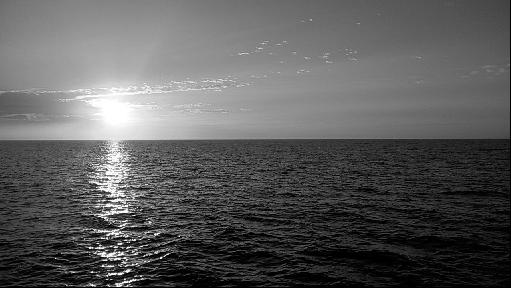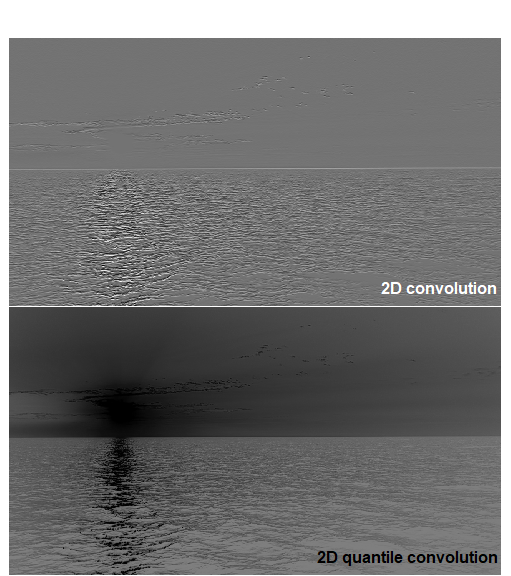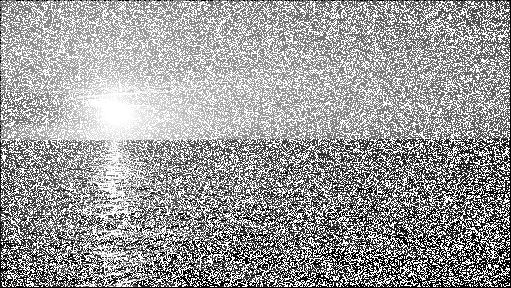# imagine

IMAGing engINE, Tools for Application of Image Filters to Data Matrices

This package was built up to take numeric data matrices and apply image-filtering algoriths.

Algoriths used by imagine include median-filter and 2D-convolution based algoriths performed on Rcpp (C++) in order to speed up the application of this filters to large numeric matrices.

## Installation

For installing imagine, as follows:

install.packages("imagine")

## Engines

imagine performs algorithms written in Rcpp (called ‘engines’), ensuring a faster application of filters. At version 1.5.0, imagine includes four main engines, described as follows:

• Engine 1: Basic 2D-convolution algorithm. It multiplies kernel by the neighborhood of each cell and then it sums all values.
• Engine 2: Make the same of Engine 1 but, at the end, it returns the position indicated by the parameter probs.
• Engine 3: It uses the radius argument to extract the values of squared neighborhood ($$radius \times radius$$) and calculates the mean.
• Engine 4: It uses the radius argument to extract the values of squared neighborhood ($$radius \times radius$$) and returns the position indicated by the parameter probs
• Engine 5: It performs a generalization of the Contextual Median Filter propose by Belkin & O’Reilly (2009). The default parameters reproduce the algorithm of the paper, but the function allows certain customization.

## Main functions

There are 5 main functions and 2 wrappers:

### Convolution functions

# Build kernels
# Kernel 1: For bottom edge recognition
kernel1 <- matrix(c(-1, -2, -1,
0,  0,  0,
1,  2,  1),
nrow = 3)

# Kernel 2: Diagonal weighting
kernel2 <- matrix(c(-2, 0, 0,
0, 1, 0,
0, 0, 2),
nrow = 3)

# Apply filters
convolutionExample  <- convolution2D(X = wbImage, kernel = kernel1)
convQuantileExample <- convolutionQuantile(X = wbImage, kernel = kernel2, probs = 0.1)

In order to compare results, we will plot both data (original and filtered) using image function, as shows in figures 1 and 2.

OriginalFigure 2: Original matrix

FilteredFigure 1: Filtered matrices

### Median-filter asociated functions

# Add some noise (NA) to the image (matrix)
set.seed(7)
naIndex <- sample(x = seq(prod(dim(myMatrix))), size = as.integer(0.4*prod(dim(myMatrix))), replace = FALSE)
myMatrix[naIndex] <- NA

# Build kernel

# Apply filters
medianfilterExample   <- medianFilter(X = myMatrix, radius = radius, times = 10)
Now, we will plot both data (original and filtered) using image function, as shows in figures 1 and 2.